## Wednesday, January 9, 2013

### Bringing Circles Together

Question: Start with two circles with radii of 2.64 (of whatever units of length you like) each.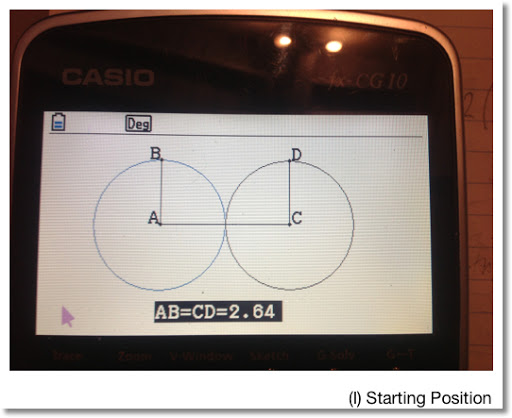Move the circle outlined in blue (on the left) to the right. How is the total area of the figure affected?

Formulas We Need:

Area of a Circle: A = π * r^2

Area of an Ellipse: A = π * a * b
Where a = length of the semi-major axis
And b = length of the semi-minor axis

The circles in (I) are joined together, each with a radius of 2.64. Calculating the total area is fairly easy:

A = 2 * π * 2.64^2 = 43.79128832 ≈ 43.79

Keep this result in mind.

Note that I will refer to each picture on this blog entry by (I), (II), (III), etc.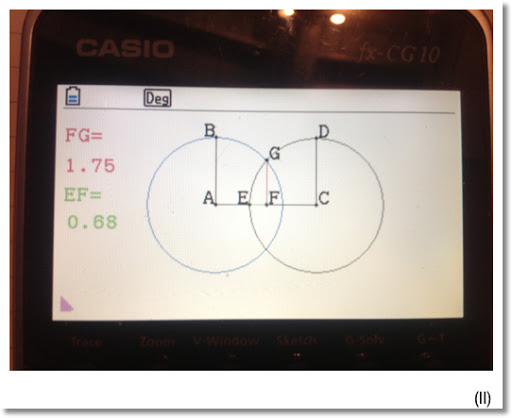Using the Geometry Mode of the Casio Prizm, I drew two circles shown in (I). Moving the blue circle to the right 10 "clicks" and taking measurements yield two overlapping circles. The total area is still the area of the circles less the overlap of the two circles. The overlap is in the form of an ellipse.

Note that line segments EF and FG represent the semi-axis of the overlap ellipse.

The measure of the segments EF and FG, according to the Prizm geometry mode, were 0.68 and 1.75 respectively.

The area of the resulting figure to (II):

A = 2 * π * 2.64^2 - π * 1.75 * 0.68 ≈ 40.05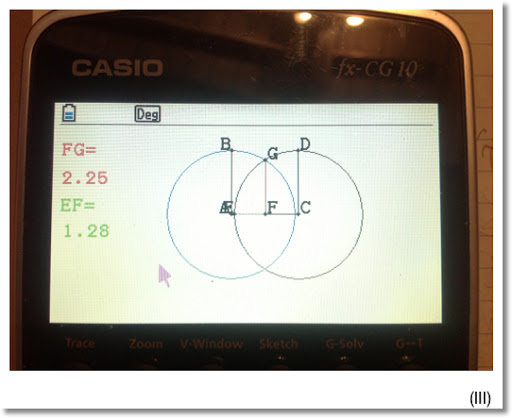At stage (III) ("20 clicks in"), EF = 1.28 and FG = 2.25. Hence the area of the figure in (III) is:

A = 2 * π * 2.64^2 - π * 1.28 * 2.25 ≈ 34.74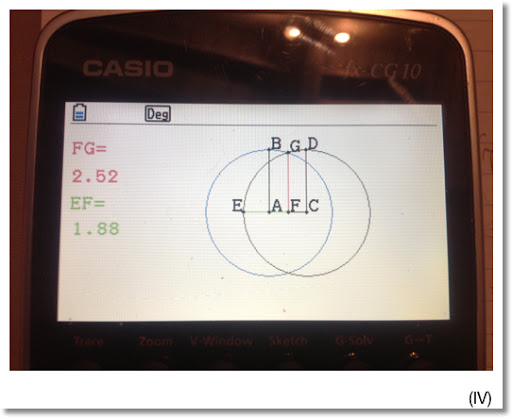At stage (IV) (30 "clicks" in), we have EF = 1.88 and FG = 2.52. Then the area is:

A = 2 * π * 2.64^2 - π * 1.88 * 2.52 ≈ 28.91.

Eventually, the circle outlined in blue meets the circle outlined in black, see (V).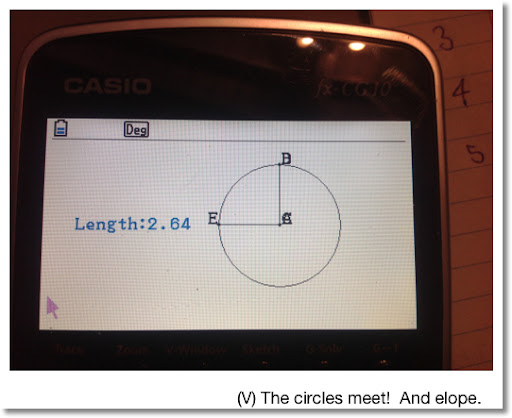The area enclosed by the shape is now A = π * 2.64^2 ≈ 21.90.

I am really enjoying the Casio Prizm. After some use and getting used to the menu structure of the calculator (this applies for most Casio graphing calculators), I find the calculator is easy to use, and I can get to where I want quickly.

Until next time, wishing you good health,

Eddie

This blog is property of Edward Shore. 2013

### Retro Review: Radio Shack EC-4026

Retro Review:   Radio Shack EC-4026 Quick Facts: Model:  EC-4026 Company:  Radio Shack Equivalent Of:  Casio fx-4500P, Casio fx-4500PA Years...